Have learned and survey the LSTM for a lone time. In this night I will give a short description about the LSTM structure itself and some applications involved.

Before the discussion, I strongly recommend people who didn’t know LSTM before reading this blog Understanding LSTM Networks, this is an explicit essay that talking about LSTM clearly, you won’t want to miss it! Anyway, I will take some contents from this blog first, to give a first sight of LSTM to you readers.

## What is LSTM? Why we need it?

I suppose you have some knowledge about neural network and some conventional models, — such as recurrent neural network. The recurrent neural network (RNN) is a architecture that can capture the context of sequence data, such as a paragraph with many sentences, or a continuous speech signals. The difference between RNN and a standard forward neural network is RNN apply both a current input information and
previous hidden state information at one step, generate a new hidden state of the current step, then pass this hidden state information to the next step, recurrently. The conventional structure of RNN (without other feature, varients) is like that: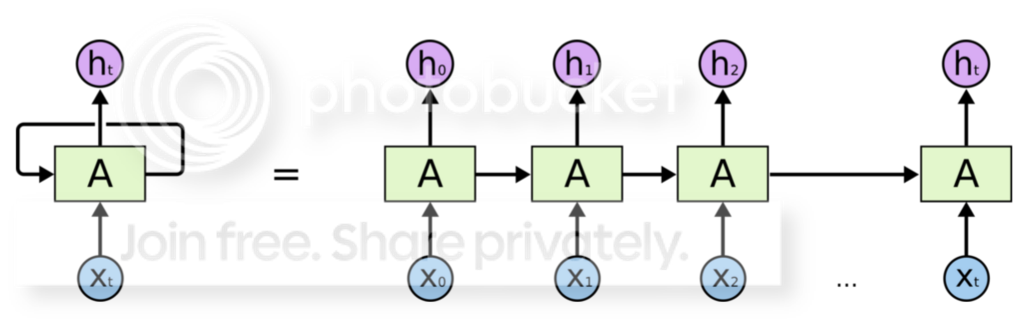and the corresponding formula is:

$h_{t} = \sigma (W_{xh}X + W_{hh}h_{t-1} + b_{bh})$

Then you can get a output from the information of $h_{t}$ by a full-connection layer, the category of output can be a real value, a softmax… well it depends on your tasks, both (or some else) is Ok.

Good, it sounds great right? Now we have a suitable model for many tasks that need to capture context, especially for NLP, the tasks such as sentiment analysis on microblog/forum/.., dialog act analysis on discourse, machine translation, language modeling.. we can use RNN anywhere. Unfortunately RNN cannot work well in the long-term dependencies situation, because it will suffer “vanishing gradient problem” and “gradients explording problem” that make RNN hard to train. The second problem is easy to solve by a “clip” approach, however the first one cannot solved with real-world tricks. If you want to explore more details about that, you can survey Ilya Sutskever’s doctorial thesis 『Training Recurrent Neural Networks』. In that thesis, the author gave a short description about “vanishing gradient”. You can just imagine during in the BPTT, the hidden weights $W_{hh}$ will multiply itself many times iteratively as the gradient propagate backwards. If the eigenvalue of hidden weights is less than 1 at some time, it will decrease to zero rapidly after some steps which means the gradients cannot propagate anymore. Therefore RNN is hard to use when the length of sequence is too long.

In order to solve this problem, researchers porposed a more complex achitecture, the Long Short-Term Memory (LSTM). Comparing wtih RNN, the LSTM has more components: input/forget/output gate, cell/hidden state that can transmit the information in multiple time scale. The input gate and forget gate is a [0,1] range sigal to controll the amount of information that the current cell genreate (which we call it “cell information candidate”) to add, and the amount of previous cell information to discard. The output gate decide how much information the hidden state will be received from the cell. You can get a more clear tutorial from the blog I mentioned at first. However the keypoint of my essay is how to use LSTM in different tasks, and corresponding variants about LSTM, also include some experimental conlcusions about inner properties. Therefore let’s get start quickly.

## Using LSTM on classification tasks

LSTM is recently used in classification tasks several times.  discussed how to use LSTM on sentence-level sentiment classification taks. They did experiments on twitter, predicted the sentiment polarity of each tweet independently. In their models, they regard the words in one sentence as a sequence. In each step, a LSTM apply both current word vector and a previous hidden vector as input, compute the current hidden state then pass it to next step. In this way, after we apply the last word of the whole sentence, we will get the last hidden state included the information of last word and all previous words, which means we can use the last hidden state to represent the sentence information. Then we can conduct the the tasks with these representations.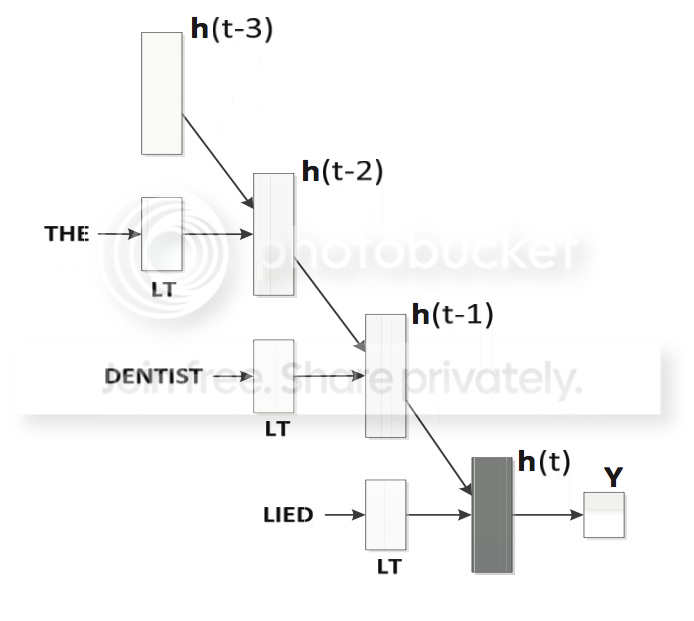In this figure, $h_{t}$ means the $t$ time step’s hidden state, while LT means lookup table, the word’s vector.Each $h_{t}$ is decided by the LT and the $h_{t-1}$ we got before. We got the sentence embedding at the end of the sentence.

Then we can use softmax to get the output predict label of each tweet.

One contribution in this paper is that author notice the LSTM can capture the word’s order in one sentence, which can somehow consider the linguistics prior knowledge of one sentence. It’s maybe the reason that LSTM is better than conventional CNN in the sentence embedding process. Another contribution is that we can tuned the word vector when training. The motivation of this change is the word’s meaning in different domain is maybe different. For example, the representation of word “good” in a normal sentence and in a “not good” phrase, is surely different, because the phrase “not good” express a negative sentiment, which may change the word’s embedding in some way.

As the paper show, we can use LSTM to generate a representation from words to sentences. So why not use the same approach in the document emedding? Like the process that generate sentence representation from a sequence words, we can also generate document representation from a sequence sentences. Therefore let us turn our sight into the second paper , which used two level LSTMs in the document level sentiment classificaion task. The architecture they used can be displayed like: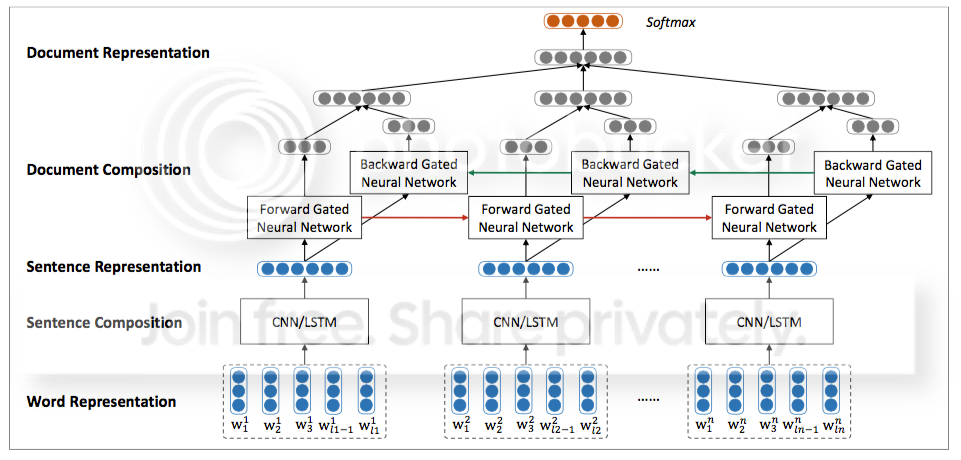The task they want to solve is document-level classification.In this paper, the sentence representation were generated by a CNN/LSTM approach, just like the last paper. What’s different is they used another LSTM to generate document vectors from these sentence embeddings. Concretely, they make two breakthroughs which acheived performance outperformed the conventional two level LSTM. First, they use a LSTM variant: Gated RNN, which the structure is more simply than LSTM while also solve the vanishing problem yet. You can gain more detail about GRU in , however I’m not decide to get more depth about GRU here.

Different with the last paper, during the document representation, they use not only the last hidden state generated, but also consider the average of all hidden states generated at each step.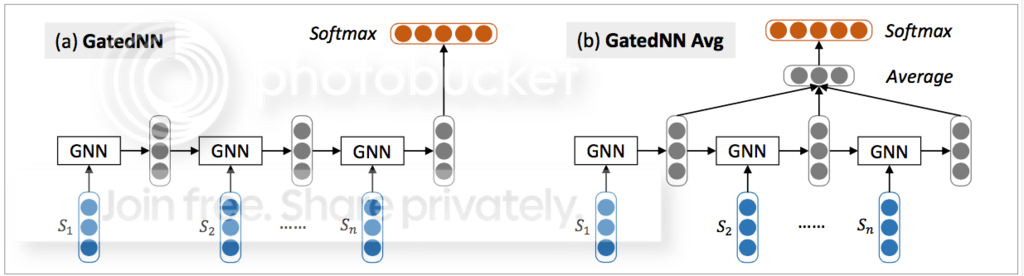Because the last hidden state is generated from both the last word input and previous hidden vector, and sometime the last word will influence the sentence representaion a lot, which means the representation cannot capture the whole sentence information properly. We want to add the influence of previous hidden vectors, so the average pooling is a effective tricks.

Another contribution is the author also used backward LSTM, and concatenate the output both from the forward LSTM and backward LSTM– we call this structure: Bidirectional LSTM. The bidirectional structure is also a convetional approach in deep learning. The motivation of this structure is in some situation the backward sequence can be also take into account in order to gain much better performance. A standrad formula about BiLSTM is showed below.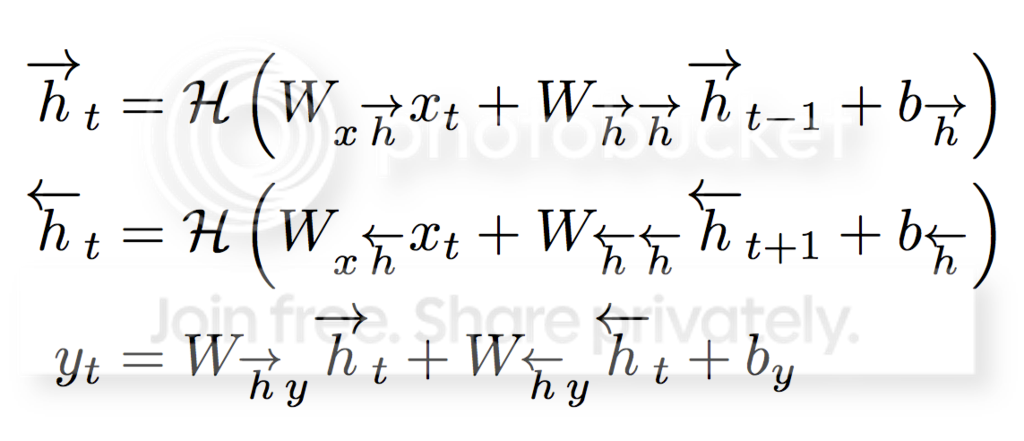with the corresponding figure: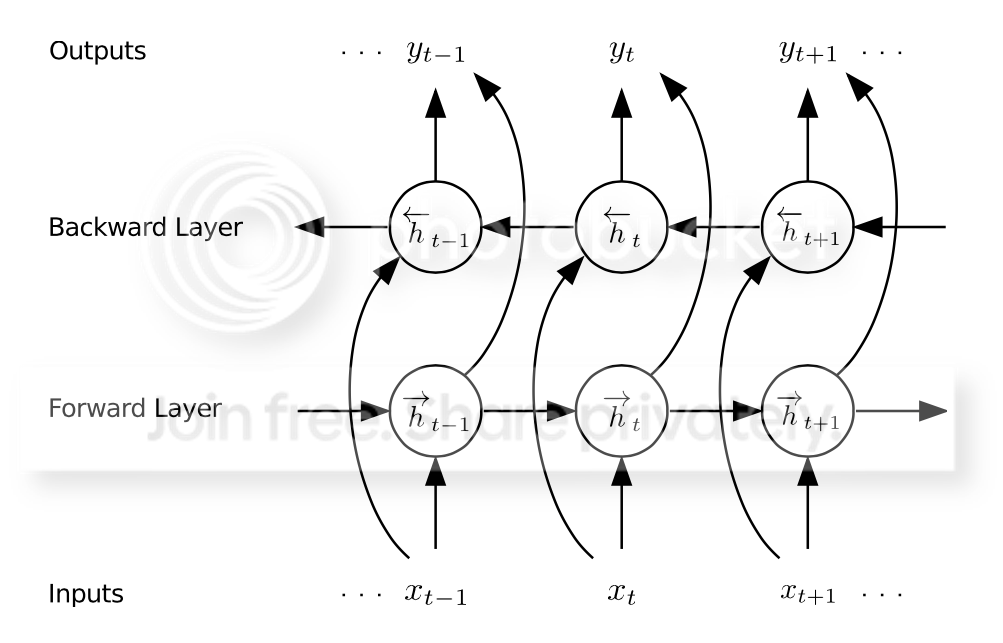Notice that the forward and backward LSTMs are not shared their weights. The output of Bi-structure is concatenate the outputs of forward and backwards. Then you can use softmax, sigmoid to get the last output value… as you like.

So far we talk about how to use LSTM in the sentence/document embedding process, the ways to get the hidden state representations are somehow different. You can choose one approach flexibly, it depends on your detail tasks. Also using LSTM in the sequence classification tasks is effectively, it can capture the context.

Then we will take a short journey on the topics about how to using LSTM on the machine translation task; how to add feartures into LSTM to adapt for different tasks; and in the end we will show another LSTM tree variant that use LSTM not in a sequence data– You can also use LSTM in the tree-based structure.

## Using LSTM on the genreration task

 give us a enlightment that how to use LSTM to do the machine translation task, a generation task in fact. This paper proposed a novel model that combined the LSTM/GRU and autoencoder. First, the encoder is an RNN that read symbol (word) of an input sequence sequentially, and the decoder is also another RNN which is trained to generate the output sequence by predicting the next symbol given the current hidden state. When the “stop symbol” has been predicted, the translate process is finished and we got a sequence symbols generated as last. The symbels we got is just the translation of original language.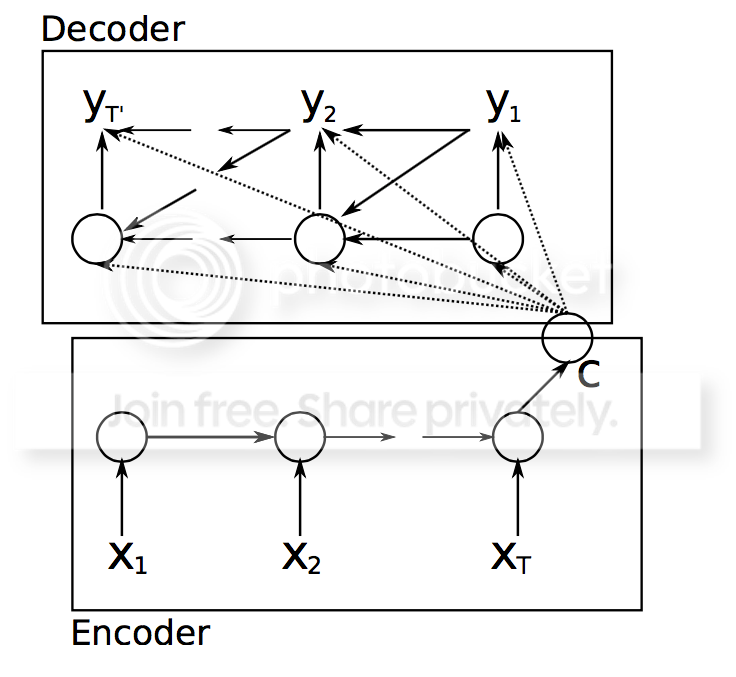Then what next we need to do is just train the RNN-encoder-decoder to minimize the objective loss.

Another generation task that used LSTM is reported by the EMNLP2015 best paper . This paper give us an important motivation that how to add “features” on the LSTM stucture. The task they conduct is given some keywords and other important information, we want to generate a sentence that include these information, meanwhile we hope the sentence is natural for human that can be understood easily. For example, in the “restaurant domain”, if we are given some key words (in this paper the keywords is denoted by DA act and slot name and value) like “Chinese food” “half price” “today”, we may want to generate a sentence like “Today the chinese food in the restarant is in half price”.

So how to use LSTM in this kind of generation tasks? An intuition is we can just use LSTM like some language modeling that predict next word distribution by current and previous word generated. The next difficulty is how to capture these keywords information during the generation process. In this paper, they use a LSTM variant: senmatic conditioned LSTM (SC-LSTM). First, they use some 1-hot vector to denote these keywords infor: if the keywords appears in a sentence, the corresponding 1-hot vector’s value is 1, otherwise is zero. Then they concatenate all these vectors to compose a length-fixed vector $d_{t}$. Next step is add the vector to the LSTM. They add another cell called “DA-cell”, which composed by a keep-variation vector $d_{t}$, a reading gate capture the multi-scale influence of the $d_{t}$ at each time.

r_{t} = \sigma(W_{wr}w_{t} + \alpha W_{hr}h_{t-1}) \\ d_{t} = r_{t} \odot d_{t-1}

Then we can add the addtional keyword information to the main fomula of cell state.

c_{t} = f_{t} \odot c_{t-1} + i_{t} \odot \tilde{c}_{t} + tanh(W_{dc}d_{t}) \\

One important tip about $d_{t}$ is we didn’t feed a different $d_{t}$ as a input in each step. We just keep one global $d_{t}$, and change its value at each step, then pass the changed $d_{t}$ to the next step. At each step, when one keyword has been predicted, the corresponding value in the $d_{t}$ will be decrease to zero. So we hope at the end of the genrate sentence, all the value in $d_{t}$ will be zero.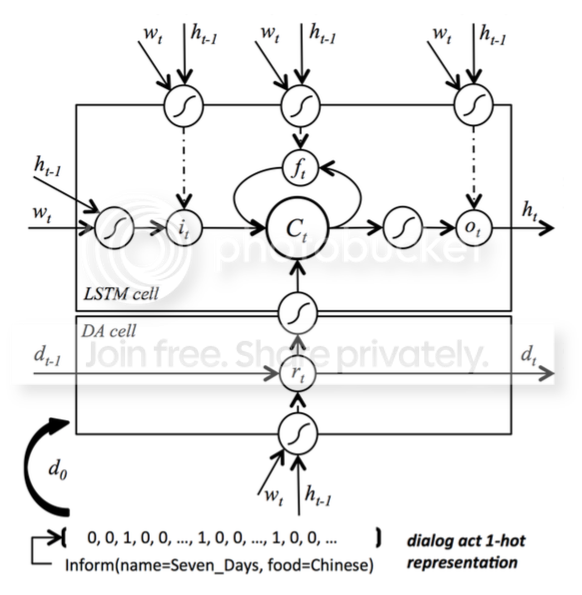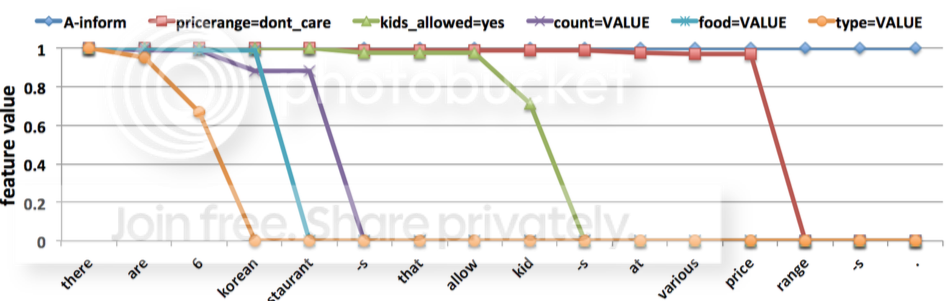(two figures shows the architecture of SC-LSTM and how the $d_{t}$ decrease when the keywords has been predicted.)

Therefore the approach in this paper propose a way to add some domain-specific features into LSTM. Of course we can also add some features to the input/forget/output gate and cell state linearly, it’s depended on your requirement.

## Tree-based LSTM

At the end of the applications section, we will introduce a specific structure about LSTM: Tree-LSTM, which can be used into some senmantic analysis task .

Imagine we now replace the orginal sequence data by a new tree-based data: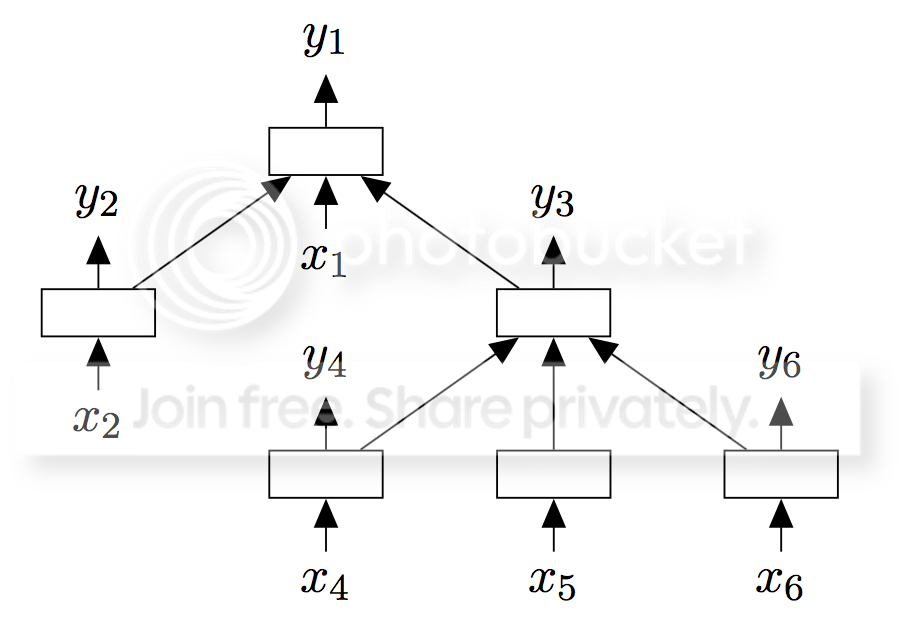That for each node which is not the leaf node in a tree, it will apply not only one $h_{t}, c_{t}$ as previous information, but in fact a set of ${h_{c_{1..k}}, c_{c_{1..k}}}$ from its $k$ children. Then normally, if we don’t care the number of children, which means each parent node can have variable-size of children, we can have LSTM formula like that: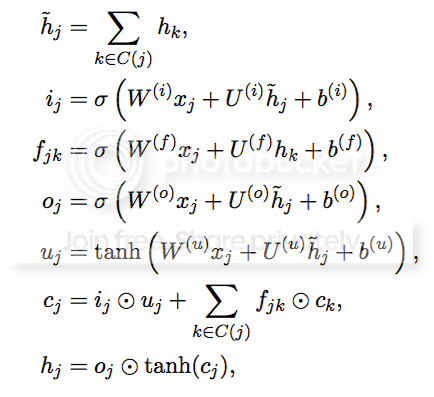Notice that only we distinguish the forget gate and corresponding cell state from each child node, because we hope considering the cell state of children respectively.

But if in our task, what we need is a fixed-size children tree, we can just distinguish each childern by all the input/forget/output gate, and also included hidden and cell state, which we call this kind of tree: N-ary Tree LSTM: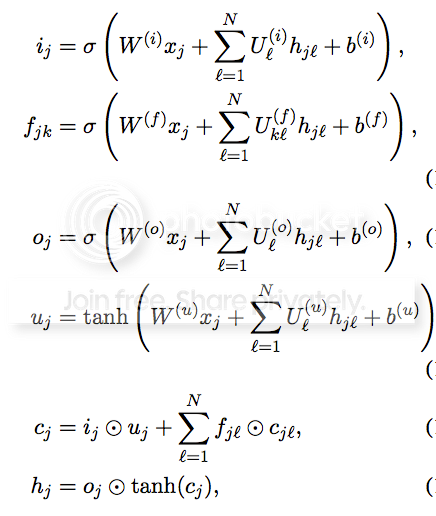Both these two Tree-LSTM can be used in the taskes that has a natural tree structure (semantic analysis of a sentence, sentiment analysis on the posts of forum, ..)

All above is what we want to talk about LSTM. However it’s just a start of our researching life, we will keep track of the LSTM later. What’more, in this essay, we only given a short introduction about how to use LSTM on the various tasks, but the analysis of LSTM itself is missed yet – for example, which parts of LSTM is more important? Which components comtribute most in the architecture? Can we remove the input gate, forget gate, output gate? Can we couple the input/forget gate, just like GRU does? The answer of these problem we will keep going on these days, and if you want to know the result immediately, you can read up the  , which may be give you a short answer.

### Reference

•  Wang, X., Liu, Y., Sun, C., Wang, B., & Wang, X. (2015). Predicting Polarities of Tweets by Composing Word Embeddings with Long Short-Term Memory.

•  Tang, D., Qin, B., & Liu, T. (2015). Document modeling with gated recurrent neural network for sentiment classificaGon. EMNLP.

•  Cho, K., Van Merriënboer, B., Gulcehre, C., Bahdanau, D., Bougares, F., Schwenk, H., & Bengio, Y. (2014). Learning phrase representations using rnn encoder-decoder for statistical machine translation

•  Wen, T. H., Gasic, M., Mrksic, N., Su, P. H., Vandyke, D., & Young, S. (2015). Semantically Conditioned LSTM-based Natural Language Generation for Spoken Dialogue Systems.

•  Tai, K. S., Socher, R., & Manning, C. D. (2015). Improved semantic representations from tree-structured long short-term memory networks.

•  Graves, A., Mohamed, A. R., & Hinton, G. (2013, May). Speech recognition with deep recurrent neural networks.

•  Chung, J., Gulcehre, C., Cho, K., & Bengio, Y. (2014). Empirical evaluation of gated recurrent neural networks on sequence modeling.

•  Greff, K., Srivastava, R. K., Koutník, J., Steunebrink, B. R., & Schmidhuber, J. (2015). LSTM: A Search Space Odyssey.

•  Jozefowicz, R., Zaremba, W., & Sutskever, I. (2015). An Empirical Exploration of Recurrent Network Architectures.